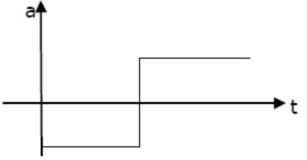# If the velocity-time graph has the shape AMB,Question:

If the velocity-time graph has the shape $\mathrm{AMB}$, what would be the shape of the corresponding acceleration-time graph?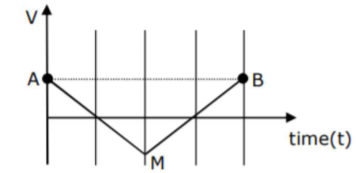1. (1)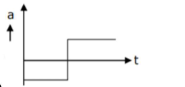2. (2)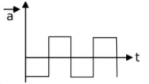3. (3)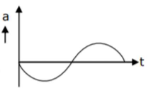4. (4)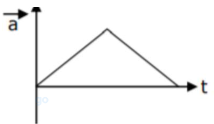Correct Option: 1

Solution: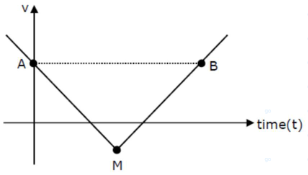$a=\frac{d v}{d t}=$ slope of $(v-t)$ curve

If $m=+$ ve, then equation of straight line isc

$y=m x+c \Rightarrow v=m t+c$

(for MB)

If $m=-$ ve, then equation of straight line is

$y=-m x+c \Rightarrow v=-m t+c($ for $A M)$

If we differentiate equation (1) and (2), we get

$\mathrm{a}_{\mathrm{MB}}=+\mathrm{ve}=\mathrm{m}$

$a_{A M}=-v e=-m$, so graph of $(a-t)$ will be